Miscellaneous

Chapter 9 Class 11 Straight Lines
Serial order wise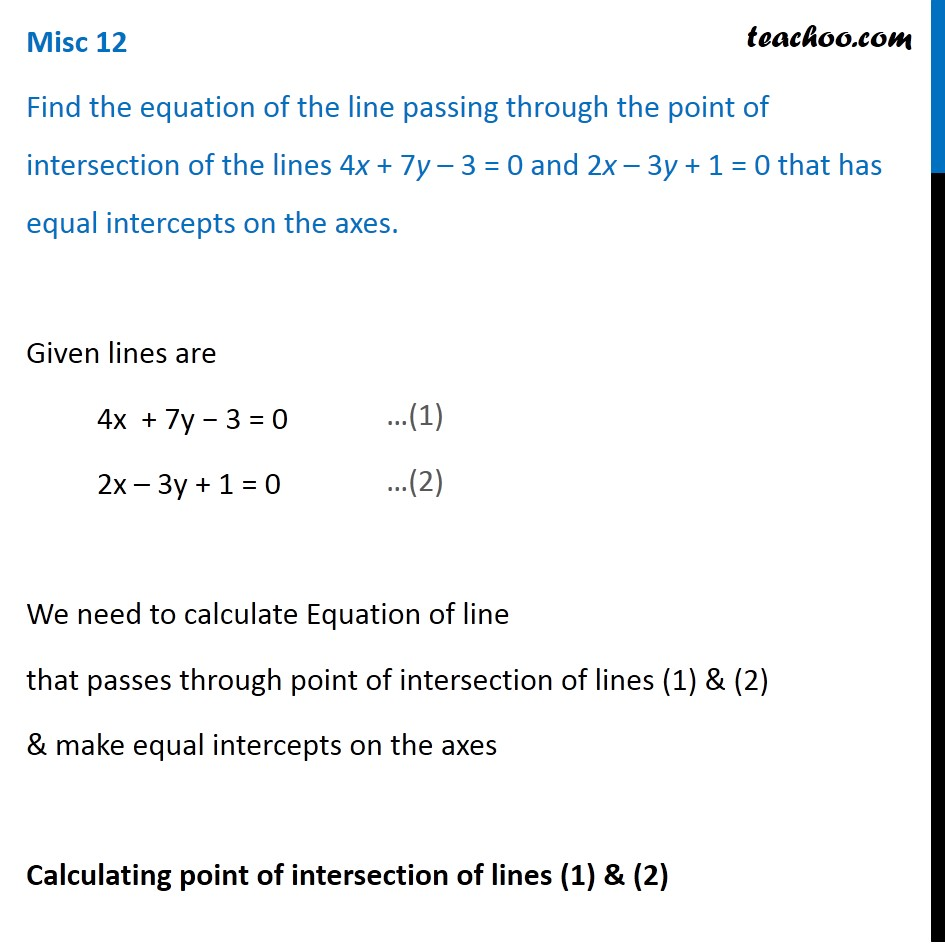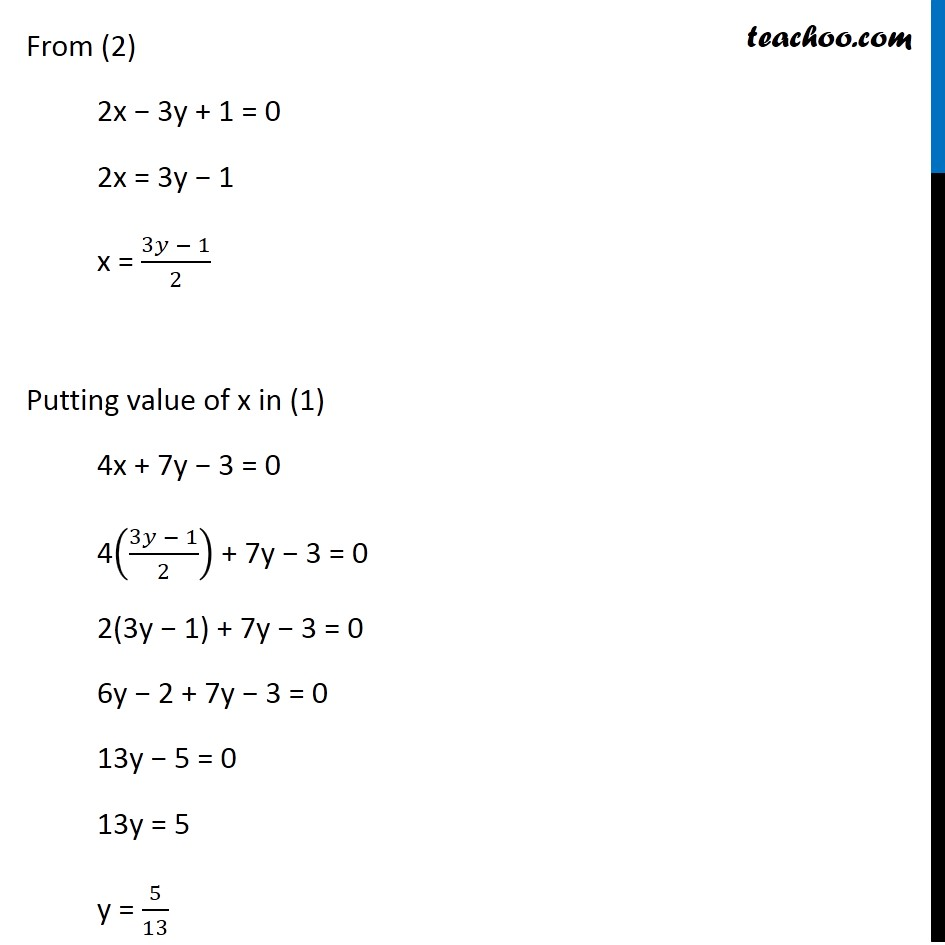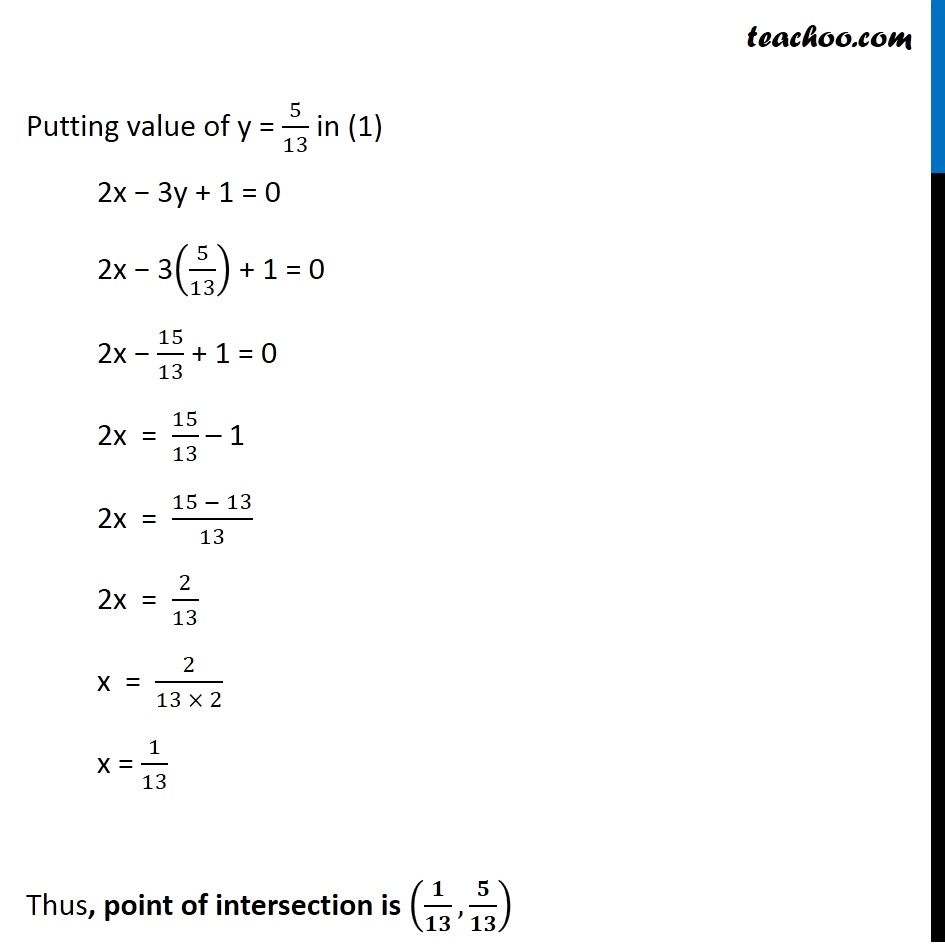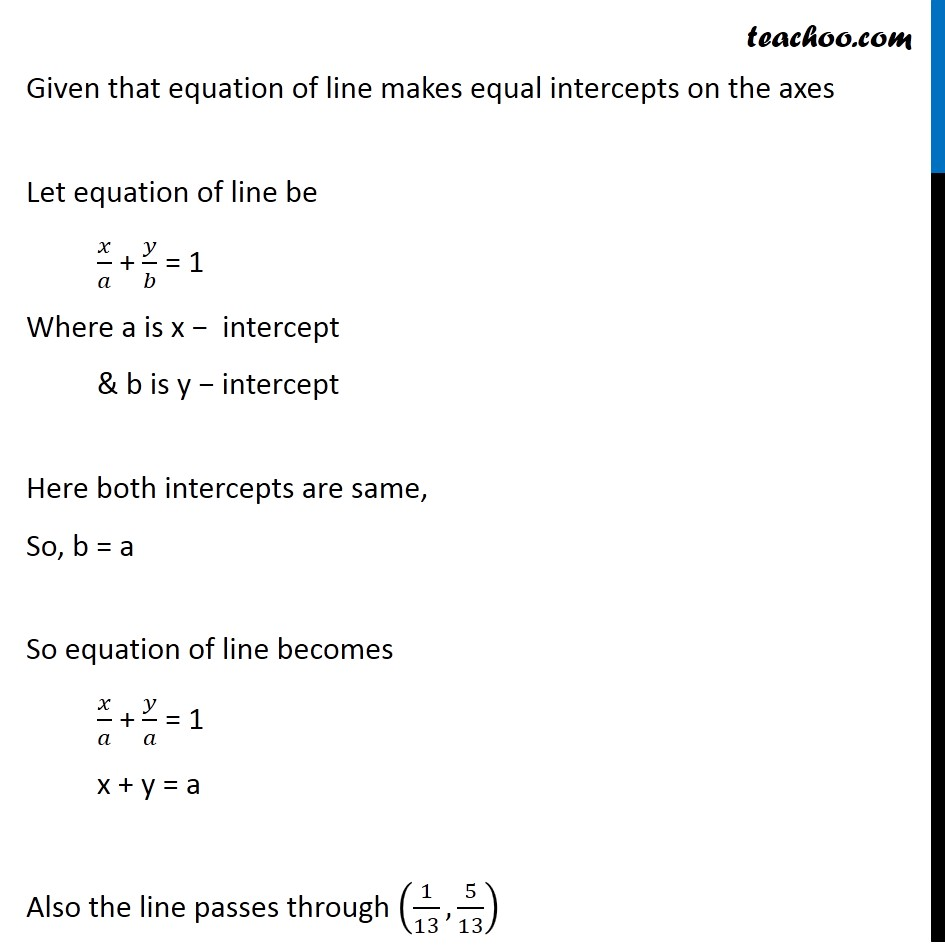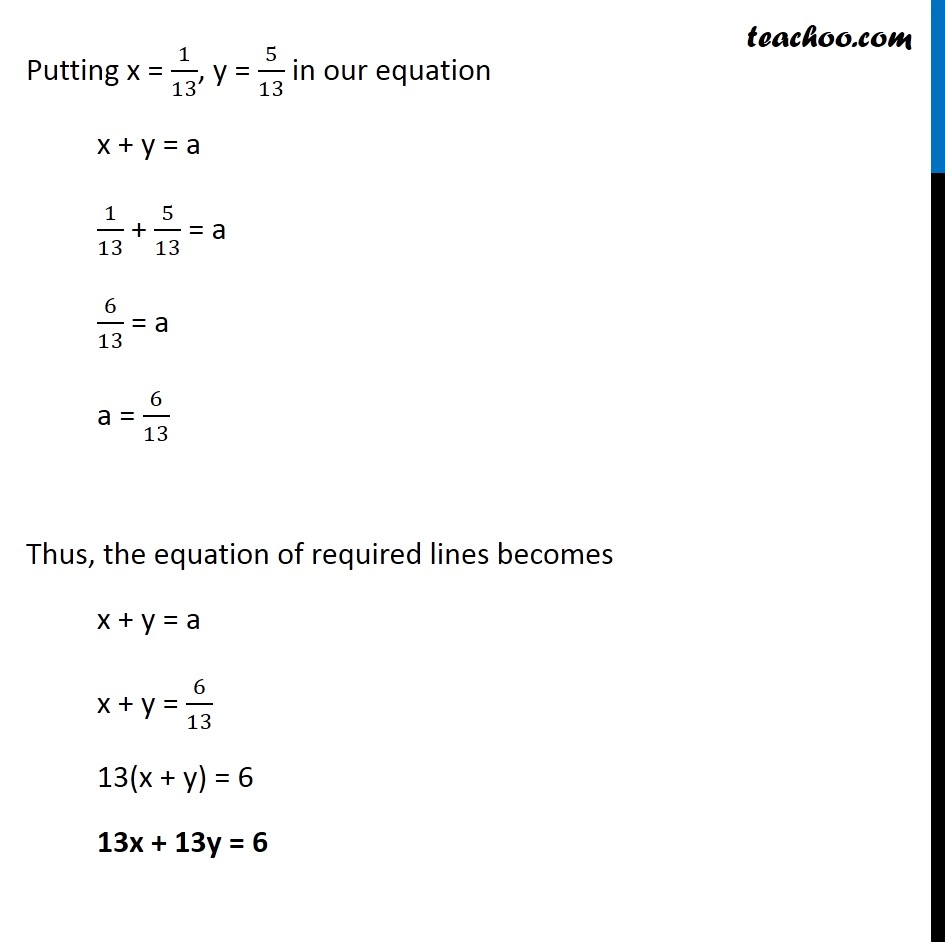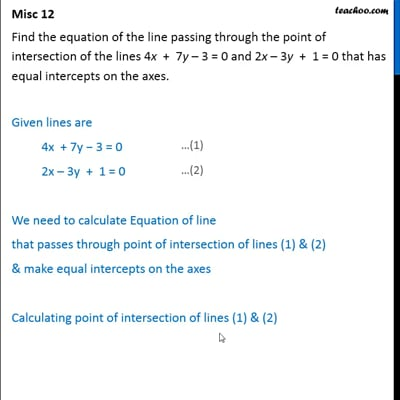This video is only available for Teachoo black users

Learn in your speed, with individual attention - Teachoo Maths 1-on-1 Class

### Transcript

Misc 11 Find the equation of the line passing through the point of intersection of the lines 4x + 7y – 3 = 0 and 2x – 3y + 1 = 0 that has equal intercepts on the axes. Given lines are 4x + 7y − 3 = 0 2x – 3y + 1 = 0 We need to calculate Equation of line that passes through point of intersection of lines (1) & (2) & make equal intercepts on the axes Calculating point of intersection of lines (1) & (2) Misc 11 Find the equation of the line passing through the point of intersection of the lines 4x + 7y – 3 = 0 and 2x – 3y + 1 = 0 that has equal intercepts on the axes. Given lines are 4x + 7y − 3 = 0 2x – 3y + 1 = 0 We need to calculate Equation of line that passes through point of intersection of lines (1) & (2) & make equal intercepts on the axes Calculating point of intersection of lines (1) & (2) Misc 11 Find the equation of the line passing through the point of intersection of the lines 4x + 7y – 3 = 0 and 2x – 3y + 1 = 0 that has equal intercepts on the axes. Given lines are 4x + 7y − 3 = 0 2x – 3y + 1 = 0 We need to calculate Equation of line that passes through point of intersection of lines (1) & (2) & make equal intercepts on the axes Calculating point of intersection of lines (1) & (2) Misc 11 Find the equation of the line passing through the point of intersection of the lines 4x + 7y – 3 = 0 and 2x – 3y + 1 = 0 that has equal intercepts on the axes. Given lines are 4x + 7y − 3 = 0 2x – 3y + 1 = 0 We need to calculate Equation of line that passes through point of intersection of lines (1) & (2) & make equal intercepts on the axes Calculating point of intersection of lines (1) & (2) Misc 11 Find the equation of the line passing through the point of intersection of the lines 4x + 7y – 3 = 0 and 2x – 3y + 1 = 0 that has equal intercepts on the axes. Given lines are 4x + 7y − 3 = 0 2x – 3y + 1 = 0 We need to calculate Equation of line that passes through point of intersection of lines (1) & (2) & make equal intercepts on the axes Calculating point of intersection of lines (1) & (2) From (2) 2x − 3y + 1 = 0 2x = 3y − 1 x = (3𝑦 − 1)/2 Putting value of x in (1) 4x + 7y − 3 = 0 4((3𝑦 − 1)/2) + 7y − 3 = 0 2(3y − 1) + 7y − 3 = 0 6y − 2 + 7y − 3 = 0 13y − 5 = 0 13y = 5 y = 5/13